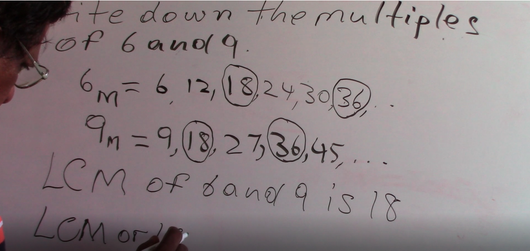Grade 6 & 7 - Multiples (LCM)

Regular price \$2.00 Sale

A 5 and 1/2 math tutorial for Grade 6 & 7 on Multiples - LCM. Tutorial done by M. Chan (Trained Certified Teacher). First part of this lesson is Multiples (Introduction).

We notice here that 18 and 18 are common multiples of 6 and 9 and also 36 and 36, but you will also notice that 18 is the lowest multiple we have of 6 and 9. We can find more multiples if we continue, between 6 and 9, but 18 as we notice, is the Lowest Common Multiple....